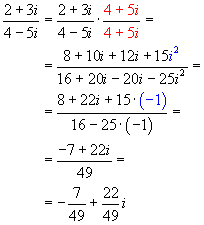Math Calculators, Lessons and Formulas

It is time to solve your math problem

mathportal.org

Complex numbers arithmetic

This solver can performs basic operations with complex numbers i.e., addition, subtraction, multiplication and division. Step-by-step explanations are provided.

Arithmetic operations with complex numbers
Basic operations explained.
show help ↓↓ examples ↓↓
Hide steps
working...
examples
example 1:ex 1:
$$\left( 2 - 4i \right) \color{red}{+} \left(5 + 3i\right)$$
example 2:ex 2:
$$\left( \frac{1}{2} - 2i \right) \color{red}{\cdot} \left(\frac{5}{2} - \frac{6}{5}i\right)$$
example 3:ex 3:
$$\frac{4-6i}{1+i}$$
example 4:ex 4:
$$\left( \frac{7}{2} - 2i \right) \color{red}{-} \left(\frac{5}{2} + 3i\right)$$

A complex numbers are of the form , a+bi where a is called the real part and bi is called the imaginary part. This text will show you how to perform four basic operations (Addition, Subtraction, Multiplication and Division):

Example: let the first number be 2 - 5i and the second be -3 + 8i. The sum is:

(2 - 5i) + (- 3 + 8i) = = ( 2 - 3 ) + ( -5 + 8 ) i = - 1 + 3 i

Subtraction:

Subtract the real parts and subtract the imaginary parts.

Example: let the first number be -3 + 7i and the second be 6 - 9i. The sum is:

(- 3 + 7i) - (6 - 9i) = = ( - 3 - 6 ) + ( 7 - ( -9 ) ) i = - 9 + 16 i

Multiplication

To multiply complex method use the FOIL method( First, Outside, Inside, and Last. )

Example: multiply 3 + 4i and 2 - 6i

First terms: 3 * 2 = 6

Outside terms: 3 * (- 6i) = -18i

Inside terms: 4i * 2 = 8i

Last terms: 4i * (-6i) = -24 * i2 = -24 (- 1) = 24

Now, combine everything together

6 - 18i + 8i + 24 = 30 - 10i.

Division

Multiply both the denominator and the numerator by the conjugate of the denominator

Example: divide 2 + 3i and 4 - 5iQuick Calculator Search